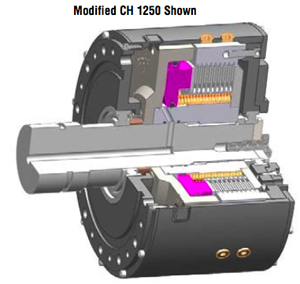# CH Technical Data for Wet or Dry Operation

## Modified Standards Available

Higher torque ranges, actuation pressures, and stronger release springs are available to meet customer’s specific design  requirements. Contact Logan for more details.Duty SF
Suggested Service Factor Table
Small Inertia Low Cycle Rate Non-pulsating Load 1.3 to 1.7
Large Inertia Low Cycle Rate Non-pulsating Load 1.7 to 2.2
Small Inertia High Cycle Rate Pulsating Load 2.2 to 3.2

*  Data shown is subject to change.  Please consult factory for current dimensional data.

### Selection Procedures

I Calculate the torque requirement for the application using one of the following formula

Torque (Lb./In.) = HP* 63025/RPM
or
Tc (Nm.) = HP* 9550/RPM

II Identify the service factor which best identifies your application from the suggested service factor table.

III Adjust the torque requirement using the selected service factor.

Gross Torque Capacity (T) / Safety Factor (SF)

T= Tc x SF or T=Tb x SF

IV. Determine if the model will:
1) Accommodate the shaft and key
2) Operate at the required speed
3) Fit within the available space

V. Determine the Drive Ring Mounting

Vl. Complete the Application Fact Sheet
provided in this brochure or complete online
at www.loganclutch.com. Call, e-mail, or

HP = Horsepower
RPM = Clutch or Brake shaft speed
WR2 = Total inertia to be stopped (Ib.ft.2)
T = Required Torque (Lb./ Ft., Nm, Lb./in)
Tc = Clutch Torque (Lb./ Ft., Nm, Lb./in)
Tb = Brake Torque (Lb./ Ft., Nm, Lb./in)
t = Time to stop (seconds)
SF = Service Factor

### Torque & Horsepower Formulas

HP = T(Lb./Ft.)*RPM /5250
Torque (Lb./Ft.) = HP*5250/RPM

HP = T(Lb./Ft.)* RPM / 63025
Torque (Lb./In.) = HP * 63025 / RPM

HP = T(Nm..) x RPM /9950
Torque (Nm.) = HP*9950/RPM

 Torque Conversion Calculators Newton meters (Nm.) to Pound inches (lb.ft.in.) Pound inches (lb.ft.in.) to Newton meters (Nm.) Newton meters (Nm.) to Pounds feet (lb. ft.) Pounds feet (lb.ft.) to Newton meters (Nm) Horsepower Conversion Calculators Horsepower (HP) to kW (Kilowatt) Kilowatt (kW) to Horsepower (HP) Pressure Conversion Calculators Bar to pounds per square inch (psi) Pounds per square inch (psi) to Bar Measurement Conversion Table Millimeters (mm) to Inches (in) Inches (in) to Millimeters (mm) Weight Conversion Table Pounds (lbs.) to Kilograms (Kg.) Kilograms (Kg.) to Pounds (lbs.) Multiplier 8.851 0.113 0.738 1.356 Multiplier .7457 1.341 Multiplier 14.5 0.068 Multiplier .03937 25.4 Multiplier 0.453 2.205